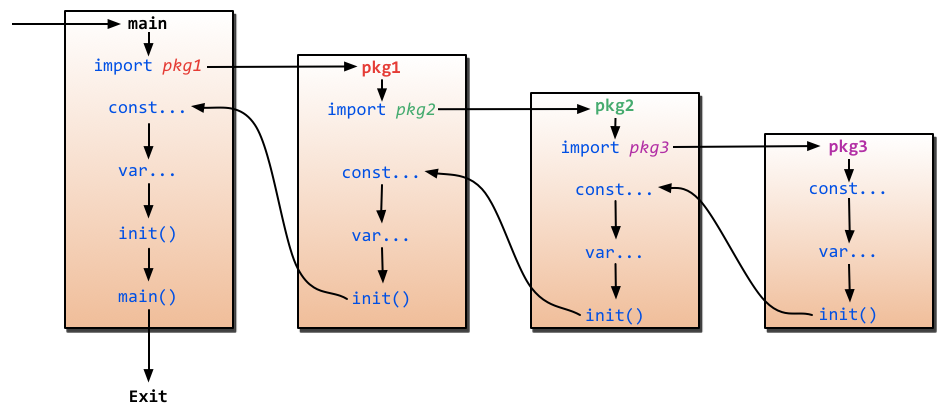# 2.3 流程和函数

## 流程控制

### if

`if`也许是各种编程语言中最常见的了，它的语法概括起来就是:如果满足条件就做某事，否则做另一件事。

Go里面`if`条件判断语句中不需要括号，如下代码所示

``````if x > 10 {
fmt.Println("x is greater than 10")
} else {
fmt.Println("x is less than 10")
}``````

Go的`if`还有一个强大的地方就是条件判断语句里面允许声明一个变量，这个变量的作用域只能在该条件逻辑块内，其他地方就不起作用了，如下所示

``````// 计算获取值x,然后根据x返回的大小，判断是否大于10。
if x := computedValue(); x > 10 {
fmt.Println("x is greater than 10")
} else {
fmt.Println("x is less than 10")
}

//这个地方如果这样调用就编译出错了，因为x是条件里面的变量
fmt.Println(x)``````

``````if integer == 3 {
fmt.Println("The integer is equal to 3")
} else if integer < 3 {
fmt.Println("The integer is less than 3")
} else {
fmt.Println("The integer is greater than 3")
}``````

### goto

Go有`goto`语句——请明智地使用它。用`goto`跳转到必须在当前函数内定义的标签。例如假设这样一个循环：

``````func myFunc() {
i := 0
Here:   //这行的第一个词，以冒号结束作为标签
println(i)
i++
goto Here   //跳转到Here去
}``````

### for

Go里面最强大的一个控制逻辑就是`for`，它即可以用来循环读取数据，又可以当作`while`来控制逻辑，还能迭代操作。它的语法如下：

``````for expression1; expression2; expression3 {
//...
}``````

`expression1``expression2``expression3`都是表达式，其中`expression1``expression3`是变量声明或者函数调用返回值之类的，`expression2`是用来条件判断，`expression1`在循环开始之前调用，`expression3`在每轮循环结束之时调用。

``````package main
import "fmt"

func main(){
sum := 0;
for index:=0; index < 10 ; index++ {
sum += index
}
fmt.Println("sum is equal to ", sum)
}
// 输出：sum is equal to 45``````

``````sum := 1
for ; sum < 1000;  {
sum += sum
}``````

``````sum := 1
for sum < 1000 {
sum += sum
}``````

``````for index := 10; index>0; index-- {
if index == 5{
break // 或者continue
}
fmt.Println(index)
}
// break打印出来10、9、8、7、6
// continue打印出来10、9、8、7、6、4、3、2、1``````

`break``continue`还可以跟着标号，用来跳到多重循环中的外层循环

`for`配合`range`可以用于读取`slice``map`的数据：

``````for k,v:=range map {
fmt.Println("map's key:",k)
fmt.Println("map's val:",v)
}``````

``````for _, v := range map{
fmt.Println("map's val:", v)
}``````

### switch

``````switch sExpr {
case expr1:
some instructions
case expr2:
some other instructions
case expr3:
some other instructions
default:
other code
}``````

`sExpr``expr1``expr2``expr3`的类型必须一致。Go的`switch`非常灵活，表达式不必是常量或整数，执行的过程从上至下，直到找到匹配项；而如果`switch`没有表达式，它会匹配`true`

``````i := 10
switch i {
case 1:
fmt.Println("i is equal to 1")
case 2, 3, 4:
fmt.Println("i is equal to 2, 3 or 4")
case 10:
fmt.Println("i is equal to 10")
default:
fmt.Println("All I know is that i is an integer")
}``````

``````integer := 6
switch integer {
case 4:
fmt.Println("The integer was <= 4")
fallthrough
case 5:
fmt.Println("The integer was <= 5")
fallthrough
case 6:
fmt.Println("The integer was <= 6")
fallthrough
case 7:
fmt.Println("The integer was <= 7")
fallthrough
case 8:
fmt.Println("The integer was <= 8")
fallthrough
default:
fmt.Println("default case")
}``````

``````The integer was <= 6
The integer was <= 7
The integer was <= 8
default case``````

## 函数

``````func funcName(input1 type1, input2 type2) (output1 type1, output2 type2) {
//这里是处理逻辑代码
//返回多个值
return value1, value2
}``````

• 关键字`func`用来声明一个函数`funcName`
• 函数可以有一个或者多个参数，每个参数后面带有类型，通过`,`分隔
• 函数可以返回多个值
• 上面返回值声明了两个变量`output1``output2`，如果你不想声明也可以，直接就两个类型
• 如果只有一个返回值且不声明返回值变量，那么你可以省略 包括返回值 的括号
• 如果没有返回值，那么就直接省略最后的返回信息
• 如果有返回值， 那么必须在函数的外层添加return语句

``````package main
import "fmt"

// 返回a、b中最大值.
func max(a, b int) int {
if a > b {
return a
}
return b
}

func main() {
x := 3
y := 4
z := 5

max_xy := max(x, y) //调用函数max(x, y)
max_xz := max(x, z) //调用函数max(x, z)

fmt.Printf("max(%d, %d) = %d\n", x, y, max_xy)
fmt.Printf("max(%d, %d) = %d\n", x, z, max_xz)
fmt.Printf("max(%d, %d) = %d\n", y, z, max(y,z)) // 也可在这直接调用它
}``````

### 多个返回值

Go语言比C更先进的特性，其中一点就是函数能够返回多个值。

``````package main
import "fmt"

//返回 A+B 和 A*B
func SumAndProduct(A, B int) (int, int) {
return A+B, A*B
}

func main() {
x := 3
y := 4

xPLUSy, xTIMESy := SumAndProduct(x, y)

fmt.Printf("%d + %d = %d\n", x, y, xPLUSy)
fmt.Printf("%d * %d = %d\n", x, y, xTIMESy)
}``````

``````func SumAndProduct(A, B int) (add int, Multiplied int) {
Multiplied = A*B
return
}``````

### 变参

Go函数支持变参。接受变参的函数是有着不定数量的参数的。为了做到这点，首先需要定义函数使其接受变参：

``func myfunc(arg ...int) {}``

`arg ...int`告诉Go这个函数接受不定数量的参数。注意，这些参数的类型全部是`int`。在函数体中，变量`arg`是一个`int``slice`

``````for _, n := range arg {
fmt.Printf("And the number is: %d\n", n)
}``````

### 传值与传指针

``````package main
import "fmt"

//简单的一个函数，实现了参数+1的操作
a = a+1 // 我们改变了a的值
return a //返回一个新值
}

func main() {
x := 3

fmt.Println("x = ", x)  // 应该输出 "x = 3"

fmt.Println("x+1 = ", x1) // 应该输出"x+1 = 4"
fmt.Println("x = ", x)    // 应该输出"x = 3"
}``````

``````package main
import "fmt"

//简单的一个函数，实现了参数+1的操作
func add1(a *int) int { // 请注意，
*a = *a+1 // 修改了a的值
return *a // 返回新值
}

func main() {
x := 3

fmt.Println("x = ", x)  // 应该输出 "x = 3"

fmt.Println("x+1 = ", x1) // 应该输出 "x+1 = 4"
fmt.Println("x = ", x)    // 应该输出 "x = 4"
}``````

• 传指针使得多个函数能操作同一个对象。
• 传指针比较轻量级 (8bytes),只是传内存地址，我们可以用指针传递体积大的结构体。如果用参数值传递的话, 在每次copy上面就会花费相对较多的系统开销（内存和时间）。所以当你要传递大的结构体的时候，用指针是一个明智的选择。
• Go语言中`channel``slice``map`这三种类型的实现机制类似指针，所以可以直接传递，而不用取地址后传递指针。（注：若函数需改变`slice`的长度，则仍需要取地址传递指针）

### defer

Go语言中有种不错的设计，即延迟（defer）语句，你可以在函数中添加多个defer语句。当函数执行到最后时，这些defer语句会按照逆序执行，最后该函数返回。特别是当你在进行一些打开资源的操作时，遇到错误需要提前返回，在返回前你需要关闭相应的资源，不然很容易造成资源泄露等问题。如下代码所示，我们一般写打开一个资源是这样操作的：

``````func ReadWrite() bool {
file.Open("file")
// 做一些工作
if failureX {
file.Close()
return false
}

if failureY {
file.Close()
return false
}

file.Close()
return true
}``````

``````func ReadWrite() bool {
file.Open("file")
defer file.Close()
if failureX {
return false
}
if failureY {
return false
}
return true
}``````

``````for i := 0; i < 5; i++ {
defer fmt.Printf("%d ", i)
}``````

### 函数作为值、类型

``type typeName func(input1 inputType1 , input2 inputType2 [, ...]) (result1 resultType1 [, ...])``

``````package main
import "fmt"

type testInt func(int) bool // 声明了一个函数类型

func isOdd(integer int) bool {
if integer%2 == 0 {
return false
}
return true
}

func isEven(integer int) bool {
if integer%2 == 0 {
return true
}
return false
}

// 声明的函数类型在这个地方当做了一个参数

func filter(slice []int, f testInt) []int {
var result []int
for _, value := range slice {
if f(value) {
result = append(result, value)
}
}
return result
}

func main(){
slice := []int {1, 2, 3, 4, 5, 7}
fmt.Println("slice = ", slice)
odd := filter(slice, isOdd)    // 函数当做值来传递了
fmt.Println("Odd elements of slice are: ", odd)
even := filter(slice, isEven)  // 函数当做值来传递了
fmt.Println("Even elements of slice are: ", even)
}``````

### Panic和Recover

Go没有像Java那样的异常机制，它不能抛出异常，而是使用了`panic``recover`机制。一定要记住，你应当把它作为最后的手段来使用，也就是说，你的代码中应当没有，或者很少有`panic`的东西。这是个强大的工具，请明智地使用它。那么，我们应该如何使用它呢？

Panic

Recover

``````var user = os.Getenv("USER")

func init() {
if user == "" {
panic("no value for \$USER")
}
}``````

``````func throwsPanic(f func()) (b bool) {
defer func() {
if x := recover(); x != nil {
b = true
}
}()
f() //执行函数f，如果f中出现了panic，那么就可以恢复回来
return
}``````

### `main`函数和`init`函数

Go里面有两个保留的函数：`init`函数（能够应用于所有的`package`）和`main`函数（只能应用于`package main`）。这两个函数在定义时不能有任何的参数和返回值。虽然一个`package`里面可以写任意多个`init`函数，但这无论是对于可读性还是以后的可维护性来说，我们都强烈建议用户在一个`package`中每个文件只写一个`init`函数。

Go程序会自动调用`init()``main()`，所以你不需要在任何地方调用这两个函数。每个`package`中的`init`函数都是可选的，但`package main`就必须包含一个`main`函数。### import

``````import(
"fmt"
)``````

``fmt.Println("hello world")``

1. 相对路径

import “./model” //当前文件同一目录的model目录，但是不建议这种方式来import

2. 绝对路径

import “shorturl/model” //加载gopath/src/shorturl/model模块

1. 点操作

我们有时候会看到如下的方式导入包

``````  import(
. "fmt"
)``````

这个点操作的含义就是这个包导入之后在你调用这个包的函数时，你可以省略前缀的包名，也就是前面你调用的fmt.Println("hello world")可以省略的写成Println("hello world")

2. 别名操作

别名操作顾名思义我们可以把包命名成另一个我们用起来容易记忆的名字

``````  import(
f "fmt"
)``````

别名操作的话调用包函数时前缀变成了我们的前缀，即f.Println("hello world")

3. _操作

这个操作经常是让很多人费解的一个操作符，请看下面这个import

``````  import (
"database/sql"
_ "github.com/ziutek/mymysql/godrv"
)``````

_操作其实是引入该包，而不直接使用包里面的函数，而是调用了该包里面的init函数。